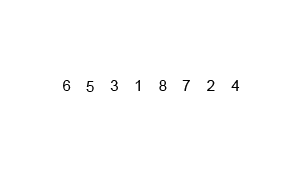### Preview lessons, content and tests

#### Computer Science & Programming solved. All in one platform.

1. To trial the platform and take tests, please take a few seconds to SIGN UP and SET UP FREE.

2. Searching for something specific? See our text overview of all tests. Scroll right for levels, and lists.

3. Student and Teacher User Guides |  Schemes of Work |   Real Teacher use Videos |

Join 36000+ teachers and students using TTIO.

### Merge Sort

Mergesort is another comparison-based algorithm also known as a "divide and conquer" algorithm and its main focus is on how to merge together two pre-sorted arrays such that the resulting array is also sorted. You may be interested to know that it was invented by the marvellous John von Neumann in 1945.

The key word of course is 'merge', so we are referring to bringing two lists together. Conceptually, a merge sort works as follows : First, divide the unsorted list into n sublists, each containing 1 element (a list of 1 element is considered sorted). Then, repeatedly merge sub lists to produce new sorted sublists until there is only 1 sublist remaining. This will be the sorted list.

>

### Challenge

#1 Write a Python program to sort a list of elements using the Merge sort algorithm

#2 Comment each line of the python solution below to show your understanding of the algorithm

### Solution

```def mergeSort(nlist):
print("Splitting ",nlist)
if len(nlist)>1:
mid = len(nlist)//2
lefthalf = nlist[:mid]
righthalf = nlist[mid:]

mergeSort(lefthalf)
mergeSort(righthalf)
i=j=k=0
while i < len(lefthalf) and j < len(righthalf):
if lefthalf[i] < righthalf[j]:
nlist[k]=lefthalf[i]
i=i+1
else:
nlist[k]=righthalf[j]
j=j+1
k=k+1

while i < len(lefthalf):
nlist[k]=lefthalf[i]
i=i+1
k=k+1

while j < len(righthalf):
nlist[k]=righthalf[j]
j=j+1
k=k+1
print("Merging ",nlist)

nlist = [14,46,43,27,57,41,45,21,70]
mergeSort(nlist)
print(nlist)```

### Animated Demowww.teachyourselfpython.com